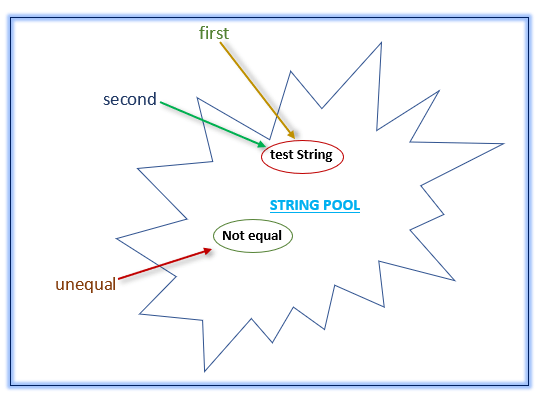## How to compare two strings in java / Various methods of string comparison in java

Comparing two strings is a commonly used operation performed by all java programmers. In fact, there would not be a single programmer who has not compared two string values. There are more than one methods of comparing strings in java. This post will cover both of them in detail.

Method 1: Using == operator
Java’s comparison operator `==` can be used to compare two string literals. It compares references and returns `true` if reference to both the strings are same, `false` if they are not. Example,

```String first = "test string"; String second = "test string"; String unequal = "not equal"; System.out.println(first == second);  // prints true System.out.println(first == third);     //prints false```

In the above example you must notice that reference variables `first` and `second` are not same(point to different strings) still their comparison returns `true`.

A string created using direct initialization is referred as String literal. Example, `String s = "codippa";`
A string created using String class constructor is a String object. Example, `String s = new String("codippa");`

Reason is JVM maintains a string pool where string values are stored. When a string literal is created, it is placed in that pool. When a new string literal is created, JVM first searches this pool. If a string with the same value is already present in the pool, then new string reference is also pointed to it as shown below.Thus, when they are compared, the result is `true`. If one of the string reference is assigned to a different value, a new string is created in the pool and the reference is updated. Now if they are compared, the result will be `false`.

Method 2: Using equals method
String class has an `equals` method which takes another string object as argument. This method when invoked on a string object compares this string object with the object supplied to it as argument. It compares
1. References of both the objects.
2. Values of both string objects.
If any of the above are same, that is, both reference variables point to same object or their values are same, then it returns `true`, `false` otherwise.
Note that `equals` method will return `true` if values of two string objects are same and even if they point to different object. Example,

```String first = "test string"; String second = new String("test string"); String unequal = "not equal"; System.out.println(first == second);  // prints false System.out.println(first.equals(second));  // prints true System.out.println(first.equals(third));   // prints false```

In the above example, `first` and `second` string reference variables point to different strings but their values are same. Thus, when compared with == operator, the result is `false` while when they are compared with `equals`, the result is `true`. Similarly, when `first` and `unequal` are compared, the result is `false` because their values are different.
Comparison using `equals` method is case sensitive. That is, test string and Test string are different values for equals. For case insensitive comparison, using `equalsIgnoreCase` method instead.
Remember that when comparing string objects using `equals`, a `null` check should be performed first. A `java.lang.NullPointerException` will be raised if the string object on which equals is called is `null`.

Method 3: Using Objects class
`java.util.Objects` class has an `equals` method which can be used to compare two strings. It takes two objects as arguments and compares them. It is similar to `equals` method in that it also compares
1. References of both the objects.
2. Values of both string objects.
If any of the above are same, it considers both the objects to be same and returns `true`. Example,

```String first = "test string"; String second =new String("test string"); System.out.println(first.equals(second)); boolean isEqual = Objects.equals(first, second); System.out.println(isEqual); // prints true```

There is no need to perform `null` check when using `Objects.equals` as it internally checks for `null` value on first object.
When to use which
1. When one string is created using direct assignment(as `String first = "test string";`) and another string is created using String class constructor(as `String second = new String("test string");`), comparison operator(`==`) will always return `false` irrespective of their values. You should use `equals` or `Objects.equals` in this case.
2. When both strings are created using String class constructor, comparison(`==`) operator will always return `false` irrespective of their values. You should use `equals` in this case too.
3. When both strings are created using direct assignment, it is better to use comparison operator(`==`).

Keep visiting for more!!!